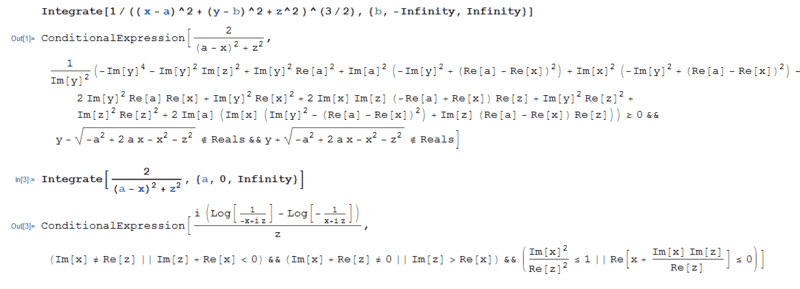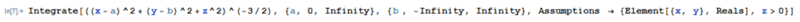# Finding electric potential using Green's function

CharlieCW

## Homework Statement

We have two semi-infinite coplanar planes defined by z=0, one corresponding to x<0 set at potential zero, and one corresponding to x> set to potential ##V_0##.

a) Find the Green function for the potential in this region
b) Find the potential ##\Phi(r)## for all points in space

## Homework Equations

Potential in terms of the Green function

$$\Phi(r)=\frac{1}{4\pi\epsilon_0}\int_V G\rho(r')dV'-\oint_S (\Phi_S \frac{\partial G}{\partial n}-G\frac{\partial \Phi_S}{\partial n})$$

Definition of the Green function

$$G(r,r')=\frac{1}{|r-r'|}+F(r,r') \ \ \ \ \ , \ \ \ \ \nabla^2 F(r,r')=0$$

$$\nabla^2 G(r,r')=-\delta(x-x')\delta(y-y')\delta(z-z')$$

Boundary conditions (Dirichlet)

$$\Phi(x<0,y,z=0)=0,\Phi(x>,y,z=0)=V_0,\Phi(r\rightarrow \infty)=0$$

## The Attempt at a Solution

Since we're free to choose our Green function as long as ##F(r,r')## obeys Laplace equation, we can choose it so that ##G=0## in ##S##. This allows us to cancel the last term inside the surface integral of the potential, so we get:

$$\Phi(r)=\frac{1}{4\pi\epsilon_0}\int_V G\rho(r')dV'-\oint_S \Phi_S \frac{\partial G}{\partial n}$$

Since we don't have a volumetric density for the first party, we can reduce this further to:

$$\Phi(r)=-\oint_S \Phi_S \frac{\partial G}{\partial n}$$

In other words, the problem has reduced to finding the appropiate Green function for the planes that satisfies the boundary conditions:

$$\Phi(x<0,y,z=0)=0,\Phi(x>0,y,z=0)=V_0,\Phi(r\rightarrow \infty)=0$$

From here on, I'm not entirely sure about my procedure. I started by solving the Green function as the solution to the equation:

$$\nabla^2 G(r,r')=-\delta(x-x')\delta(y-y')\delta(z-z')$$

with the boundary condition ##G=0## for ##z=0##. The Green function of this plane is equivalent to the potential of a charge placed near a grounded surface, which is a well known solution:

$$G(r,r')=\frac{1}{4\pi}(\frac{1}{\sqrt{(x-x')^2+(y-y')^2+(z-z')^2}}-\frac{1}{\sqrt{(x-x')^2+(y-y')^2+(z+z')^2}})$$

Which we can confirm indeed satisfies ##G=0## for ##z=0##.

However, this Green function doesn't satisfy the boundary conditions for my problem, so I don't know how to modify or find the proper Green function. I've tried test functions or even separating the Green function in parts (through a dirac delta), but nothing seems to work so far.

Once I find the Green function I can easily substitute into the equation for the potential and solve it without problem, so my real issue is finding the appropiate Green function.

Last edited:

Homework Helper
Gold Member
with the boundary condition ##G=0## for ##z=0##. The Green function of this plane is equivalent to the potential of a charge placed near a grounded surface, which is a well known solution:

$$G(r,r')=\frac{1}{4\pi}(\frac{1}{\sqrt{(x-x')^2+(y-y')^2+(z-z')^2}}-\frac{1}{\sqrt{(x-x')^2+(y-y')^2+(z+z')^2}})$$

Which we can confirm indeed satisfies ##G=0## for ##z=0##.

However, this Green function doesn't satisfy the boundary conditions for my problem
Doesn't the fact that ##G=0## for ##z=0## mean that ##G## satisfies the necessary boundary condition? Is there some other boundary condition for ##G## ?

CharlieCW
Doesn't the fact that ##G=0## for ##z=0## mean that ##G## satisfies the necessary boundary condition? Is there some other boundary condition for ##G## ?

You're right, I was confusing the boundary conditions of the potential with the ones of my Green function. However, would the Green function be the same even if the two semi-infinite planes are at different potentials? I understand the Green function must vanish on the surface, but I'm not sure if this is true even if some regions of the surface are at different potentials.

On the other hand, continuing on the problem, I found the normal partial derivative for ##z>0##:

$$\frac{\partial G}{\partial n}=-\frac{1}{2\pi}\frac{z}{(x-x')^2+(y-y')^2+z^2)^{3/2}}$$

Substituting in the equation for the potential, I get:

$$\Phi(r)=\frac{z}{2\pi}\int_{-\infty}^{\infty}dx'\int_{-\infty}^{\infty}dy' \frac{\Phi_S}{(x-x')^2+(y-y')^2+z^2)^{3/2}}$$

Now here I apply the boundary condition by setting that ##\Phi_S=V_0## for ##x>0## and zero otherwise. This allows me to replace the above integral to:

$$\Phi(r)=\frac{V_0z}{2\pi}\int_{0}^{\infty}dx'\int_{-\infty}^{\infty}dy' \frac{1}{(x-x')^2+(y-y')^2+z^2)^{3/2}}$$

However this expression has no analytical solution, so I think I'm either doing something wrong or I must modify my limits to account for a semi-infinite plane. I also tried solving it by passing to polar coordinates:

$$\Phi(r)=\frac{V_0z}{2\pi}\int_{-\pi /2}^{\pi /2}d\theta'\int_{0}^{\infty}d\rho' \frac{\rho}{(x-x')^2+(y-y')^2+z^2)^{3/2}}$$

Which after solving gives me ##\Phi(r)=V_0/2## for ##x>0##, which doesn't depend on z. However, this clearly doesn't make sense (or is incomplete) in the region near ##x=0##, since it's clear the lines from the plane from the right side (at potential ##V_0##) must connect towards the plane on the right side (at potential zero).

In other words, how do I account for the connection of the potential between the two planes on the vecinity of ##x=0##.

Homework Helper
Gold Member
You're right, I was confusing the boundary conditions of the potential with the ones of my Green function. However, would the Green function be the same even if the two semi-infinite planes are at different potentials? I understand the Green function must vanish on the surface, but I'm not sure if this is true even if some regions of the surface are at different potentials.
Yes, the Green function would be the same for any specification of ##\Phi## on the plane ##z = 0##.

On the other hand, continuing on the problem, I found the normal partial derivative for ##z>0##:

$$\frac{\partial G}{\partial n}=-\frac{1}{2\pi}\frac{z}{(x-x')^2+(y-y')^2+z^2)^{3/2}}$$

Substituting in the equation for the potential, I get:

$$\Phi(r)=\frac{z}{2\pi}\int_{-\infty}^{\infty}dx'\int_{-\infty}^{\infty}dy' \frac{\Phi_S}{(x-x')^2+(y-y')^2+z^2)^{3/2}}$$

Now here I apply the boundary condition by setting that ##\Phi_S=V_0## for ##x>0## and zero otherwise. This allows me to replace the above integral to:

$$\Phi(r)=\frac{V_0z}{2\pi}\int_{0}^{\infty}dx'\int_{-\infty}^{\infty}dy' \frac{1}{(x-x')^2+(y-y')^2+z^2)^{3/2}}$$
This all looks good. I put the integral into Mathematica, and it gave a fairly simple result.

I also tried solving it by passing to polar coordinates:

$$\Phi(r)=\frac{V_0z}{2\pi}\int_{-\pi /2}^{\pi /2}d\theta'\int_{0}^{\infty}d\rho' \frac{\rho}{(x-x')^2+(y-y')^2+z^2)^{3/2}}$$
I'm not sure how you are setting this up in polar coordinates.

CharlieCW
Yes, the Green function would be the same for any specification of ##\Phi## on the plane ##z = 0##.

Thank you for clearing this up. It makes sense too, since the Green function is purely geometrical.

However, when I asked the assistant of the course, he told me the Green function must also satisfy the boundary conditions of the potential in the first place, so the one I wrote is wrong (I showed my Green function and he told me I'm still missing some terms). I found this very confusing, as it contradicts what I've read (that the Green function must be ##G=0## on the boundary, regardless of its value). What do you think?

This all looks good. I put the integral into Mathematica, and it gave a fairly simple result.

I also tried using Mathematica to solve this expression and evaluate the limits (by setting x' as a, y' as b), and it gave me the following:Which is quite a complicated conditional expression.

I'm not sure how you are setting this up in polar coordinates.

For this I imagine a circle of radius ##\rho## which expands from the origin of coordinates all the way to infinity. To limit myself to the region of ##x>0##, I integrate from ##-\pi /2## to ##\pi /2## (the semicircle to the right). The denominator reduces to ##(x-x)^2+(y-y')^2+z^2=\rho^2+z^2##

#### Attachments

Homework Helper
Gold Member
However, when I asked the assistant of the course, he told me the Green function must also satisfy the boundary conditions of the potential in the first place, so the one I wrote is wrong (I showed my Green function and he told me I'm still missing some terms). I found this very confusing, as it contradicts what I've read (that the Green function must be ##G=0## on the boundary, regardless of its value). What do you think?
I'm certainly no expert on Green functions. But, I grabbed my copy of Jackson (2nd edition) to review the topic. He mentions that you can choose the function ##F(r, r')## so that ##G## is zero on the bounding surface so that you have "Dirichlet" boundary conditions. This seems to me to be what you want to do since you have a problem where ##\Phi## is specified on the surface. So, I don't know what the assistant of the course is referring to. I hope I'm not misleading you.

I also tried using Mathematica to solve this expression and evaluate the limits (by setting x' as a, y' as b), and it gave me the following:

View attachment 238536

Which is quite a complicated conditional expression.
It will help if you let Mathematica know that all of your parameters are real. So, you can try adding an "Assumptions" option in your Integrate command:Here, I specified ##x## and ##y## as real. Also that ##z>0##, which implies that ##z## is real and that we are avoiding ##z = 0## which would put us right on the plane. I don't know Mathematica that well, so there could be a nicer way to do this.
For this I imagine a circle of radius ##\rho## which expands from the origin of coordinates all the way to infinity. To limit myself to the region of ##x>0##, I integrate from ##-\pi /2## to ##\pi /2## (the semicircle to the right). The denominator reduces to ##(x-x)^2+(y-y')^2+z^2=\rho^2+z^2##
The limits of integration are going to be more complicated. You are integrating with respect to the primed ##x'## and ##y'##. You have taken ##\rho^2 =(x-x')^2+(y-y')^2##. The presence of the unprimed ##x## and ##y## complicates things. The origin of your ##\rho## is at the point ##(x, y)## which is not generally at the boundary of the two half-plates.

#### Attachments

•CharlieCW
CharlieCW
I'm certainly no expert on Green functions. But, I grabbed my copy of Jackson (2nd edition) to review the topic. He mentions that you can choose the function ##F(r, r')## so that ##G## is zero on the bounding surface so that you have "Dirichlet" boundary conditions. This seems to me to be what you want to do since you have a problem where ##\Phi## is specified on the surface. So, I don't know what the assistant of the course is referring to. I hope I'm not misleading you.

Thank you for your time, I really appreciate it. Indeed I also checked from Jackson and Greiner and I read that I was free to choose ##F(r,r')## so that ##G(r,r')## is zero on the surface. After a couple of exchanges it turns out we were right: the Green function only depends on the geometry of the problem. It was actually a mistake on behalf of the assistant who answered me in a hurry.

It will help if you let Mathematica know that all of your parameters are real. So, you can try adding an "Assumptions" option in your Integrate command:
View attachment 238543

Here, I specified ##x## and ##y## as real. Also that ##z>0##, which implies that ##z## is real and that we are avoiding ##z = 0## which would put us right on the plane. I don't know Mathematica that well, so there could be a nicer way to do this.

Thanks, I didn't know you could add assumptions to the integration, but it indeed after adding them it simplified a lot the solution:

$$Int(r)=\frac{1}{z}(\pi+2Arctan(\frac{x}{z}))$$

And substituting into the potential:

$$\Phi(r)=\frac{V_0 z}{2\pi}Int(r)=\frac{V_0}{2}(1+\frac{1}{\pi}Arctan(\frac{x}{z}))$$

Which now makes sense, as when ##x\rightarrow \infty## the second term vanishes and we recover the potential of an infinite plane, yet when it is close to zero we indeed get a complex behavior near the boundary of the two planes. Therefore, I think the problem is solved.

Homework Helper
Gold Member
$$\Phi(r)=\frac{V_0 z}{2\pi}Int(r)=\frac{V_0}{2}(1+\frac{1}{\pi}Arctan(\frac{x}{z}))$$
I think you dropped a factor of 2 in the last term. Otherwise, looks good to me.

Which now makes sense, as when ##x\rightarrow \infty## the second term vanishes and we recover the potential of an infinite plane, yet when it is close to zero we indeed get a complex behavior near the boundary of the two planes. Therefore, I think the problem is solved.
It is interesting that the equipotential surfaces are flat planes defined by ##z = cx##, where c is a constant. The electric field lines appear to be circular.